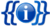# Template:Resize

{{{1}}}

 [🔄Purge]Template documentation
It is to change the font size of a text string.

## Usage

• `{{resize|N|text}}`N specifies a size, such as "95%", "1.2em", etc.
• `{{resize|text}}` — If N is omitted, as in the second line above, a default 90% size is applied.
• `{{resize|div=yes|text}}` — As above but uses a div tag instead of a span tag.

### Beware

Beware that if `text` happens to contain an equality sign (`=`), it will fail to render properly. Here are the workarounds:

1. Replace all instances of `=` with `{{=}}`, or
2. Specify `{{resize|''N''|'''2='''''text''}}` or `{{resize|'''1='''''text''}}`.

Also it will fail to wrap multiple blocks, such as multiple paragraphs or multiple bullet points. Here are the workarounds:

1. Use, for example,
`<div style="font-size:90%;">`
...multiple paragraphs...
`</div>`, or
2. Apply this template separately to each block of paragraph, bullet point etc.
3. Use {{resize|div=yes}}.
4. Use {{smalldiv}}.

## Examples

Code Result
`{{resize|This text defaults to 90% normal size.}}` This text defaults to 90% normal size.
`{{resize|80%|This text is 80% normal size.}}` This text is 80% normal size.
`{{resize|1.2em|This text is 0.2em larger than normal.}}` This text is 0.2em larger than normal.
`{{resize|We know 1+1=2 and 2+2=4. It will fail to render}}` {{{1}}}
`{{resize|We know 1+1{{=}}2 and 2+2{{=}}4.}}` We know 1+1=2 and 2+2=4.
`{{resize|1=We know 1+1=2 and 2+2=4.}}` We know 1+1=2 and 2+2=4.
`{{resize|120%|2=We know 1+1=2 and 2+2=4. This text is 120% normal size.}}` We know 1+1=2 and 2+2=4. This text is 120% normal size.

## TemplateData

```{
"description": "It is to change the font size of a text string. Two forms {{resize|<size>|<text>}} and {{resize|<text>}} are possible.",
"params": {
"1": {
"label": "Size/Text",
"description": "Specifies a css font size e.g. '80%', '1.2em', 'x-large', 'larger'. In the one parameter version the first parameter is the text and the font size is '90%'.",
"type": "string",
"required": true
},
"2": {
"label": "Text when size is given",
"description": "The text to display in the two parameter version.",
"type": "string",
"required": false
},
"div": {
"label": "Use div tag",
"description": "Set to yes to use a div instead of a span tag.",
"type": "string",
"required": false
}
}
}
```